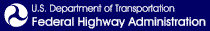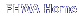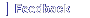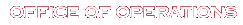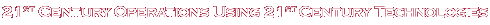# Application of Travel Time Data and Statistics to Travel Time Reliability Analyses: Handbook and Support Materials

## Appendix A. Steps and Python Code for Reliability Data Processing

This appendix presents the procedures and example Python code that can be used to process travel time data for the purpose of constructing reliability measures.

### Data Processing for Reliability

#### Data Extraction and QC

1. Determine the candidate facilities.
2. Identify traffic message channels (TMC) that are associated with the facility: One way is to obtain the TMC definition file associated with the facility, plot the TMC locations (latitude/longitude) using a geographic information system (GIS) program, and visually identify the TMCs that are located between the starting and ending points of the facility.
3. Obtain the probe data by providing the geographical limits of the facility.
4. Refine the facility data by subsetting the raw data based on desired TMCs.
##### QC
1. Review data documents.
1. Data structure and field data types:
1. The data documents provide data specifications, including description of the data columns, data types, and data lengths, as well as how raw data is collected and processed.
2. This information is critical for understanding the data characteristics and quality, for choosing the data columns to be included in the analysis, and for determining how data is imported.
2. Probe data time interval:
1. Data time interval not only determines the datetime dimension of the data, but also helps to identify data gaps and detect irregular data points along the datetime dimension.
3. If data gaps exist or imputed, data are used to fill gaps.
4. Built-in quality control (QC) mechanism from the data source, e.g., detector health and percent of imputed data from PeMS detector data.
2. Prepare summary statistics of key variables.
• Some of the summary statistics include:
• Mean, standard deviation, and range
• Number of values, number of null values
• Number of distinct values
• For the “speed” field, the summary statistics (i, ii and iii) can be produced on the entire dataset (facility), by facility direction, by TMC and by TMC and date/time.
• For “measurement_tstamp,” summary statistics ii and iii can be derived to understand if any duplicates or missing data exists.
3. Identify data gaps.
• Missing/null values
• Missing certain TMC/date/time interval combinations

Based on the nature and extent of the gaps, the following decisions can be made:

• Whether the data is usable or not
• If the data can be used, then if the data can be used as is or the gaps should be filled.
• If the gaps need to be filled, then what strategies should be employed to fill the gaps.
4. Identify errors.
• Data that are not physically possible, e.g., speed = −5 mph
• Data that are not realistic, e.g., speed > 150 mph
• Data that are significantly different from rest of the dataset, e.g., data during incident

Based on the actual cases, decisions can be made as whether the errors should be excluded or adjusted.

5. Detect other data issues:
• Duplicate observations
• Illogical TMC/date/time interval (e.g., datetime values can be problematic because of importing with incorrect format)
6. Visualize variable distributions:
• Common sense check
• Can detect directional issues

#### Spatial and Temporal Aggregation: Development of the Travel Time Distribution

##### Probe Data

The probe snapshot method uses probe data and is based on the SHRP 2 L03 method, which develops the 5-minute facility travel times by summing up all section travel times along the facility at each given time interval.24 The facility travel times can be adjusted based on the ratio of sum of the section lengths and facility length if missing section data exists. Facility-level space-mean speeds can be derived from the facility travel times.

The probe virtual method relies on an algorithm that synthesizes travel times by simulating vehicles on the time/space diagram developed from probe data. A vehicle’s speed at any given moment is determined by what link it is on at a given time. As it takes time for a vehicle to travel to a specific section, the traffic condition on that section could change by the time the vehicle arrives. In this way, end-to-end travel times are created and compiled into a travel time distribution from which reliability measures are calculated. The following figures contain the Python code for conducting reliability analyses.

##### Data Transformation: Produce “Clean” Section-Level Data.
1. Read the extracted National Performance Management Research Data Set (NPMRDS) speed data:
• Import “tmc_code,” “measurement_tstamp” and “speed” columns.
• Specify the “tmc_code” type as string, and “speed” type is float.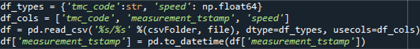Figure 34. Screenshot. Code for reading National Performance Management Research Data Set data.

df_types = {'tmc_code'; str, 'speed'; np,float64} \\ df_cols = {'tmc_code', 'measurement_tstamp', 'speed} \\ df = pd.read.csv('%s/%s' %(csvfolder, file), dtype=df_types, usecols=df_cols) \\ df['measurement_tstamp'] = pd.to.datetime(df['measurement_tstamp'])
• Find distinct “tmc_code” and “measurement_tstamp” combinations to check and remove if duplicates exist.
• Find distinct “tmc_code” of the facility.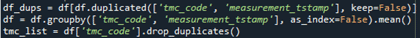Source: FHWA.

Figure 35. Screenshot. Code for reading traffic message channel data.

2. Read the NPMRDS TMC definition file:
• Subset the dataset based on the distinct “tmc_code” identified in the previous step.
• Sort the dataset by “road_order.”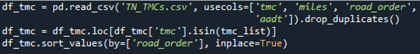Source: FHWA.

Figure 36. Screenshot. Code for reading traffic message channel definition data.

3. Create a data template by using the number of unique TMCs and all the time intervals as dimensions:
• The TMC dimension is the set of the distinct “tmc_code.”
• The datetime dimension is based on the starting/ending datetime and the time interval.
• Create the template with the dimension as the Cartesian product of the TMC and datetime dimension.
• The template does not include any data.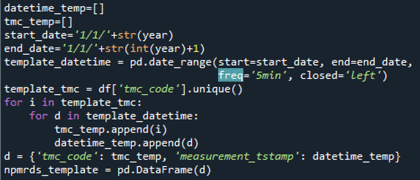Source: FHWA.

Figure 37. Screenshot. Code for creating the data template.

datetime_temp=[] \\ tmc_temp=[] \\ start_date ='1/1/'+str(year) \\ end_date='1/1'+str(int(year)+1) \\ template_datetime = pd.date_range(start=start_date, end=end_date, freq = '5min', closed='left) \\ template_tmc = df['tmc_code'].unique() \\ for i in template_tmc: \\ for d in template_datetime: \\ tmc_temp.append(i) \\ datetime_temp_append(d) \\ d = {tmc_code: tmc_temp, 'measurement_tstamp': datetime_temp} \\ npmrds_template = pd.DataFrame(d)
4. Merge the NPMRDS speed data to the template:
• Merge the template and NPMRDS data based on “tmc_code” and “measurement_tstamp” fields.
• The merge is a left join that joins the NPMRDS data to the template, keeping the dimension of the template.
• The new dataset (data) has a large dimension comparing to the original NPMRDS data and data gaps (“tmc_code” and “measurement_tstamp” without “speed”) are introduced in the merging process.
• Sort the data by “tmc_code” and “measurement_tstamp.”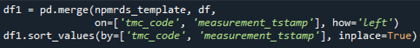Source: FHWA.

Figure 38. Screenshot. Code for Reading Merging traffic message channel and Travel Time Data.

5. Fill gaps in the merged dataset using interpolation:
• Investigate the nature and extent of the data gaps in data.
• Since the probe virtual method depends upon no gaps both temporally and geographically to simulate the vehicle movements, gaps are filled if this method is to be performed.
• Fill gaps using interpolation with appropriate method (e.g., linear, nearest, etc.).
• Perform an additional QC check to make sure all gaps have been filled.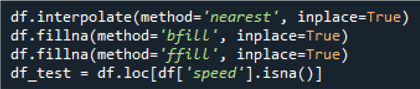Source: FHWA.

Figure 39. Screenshot. Code for data quality control check.

6. Additional datetime preparations from data, for use in aggregation step:
• Produce “day of the week” field to distinguish between weekdays and weekends.
• Produce “hour” field to define peak periods.
• Produce “date” field to define holidays.
• Produce “time” field as the time of the day dimension.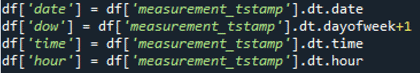Source: FHWA.

Figure 40. Screenshot. Code for creating additional temporal variables.

7. Calculate section travel time:
• Merge the data and TMC definition based on “tmc_code” field.
• The merge is a left join that gives the data the “miles,” “road_order” and “aadt” fields.
• Calculate the section travel time based on section length (“miles”) and speed.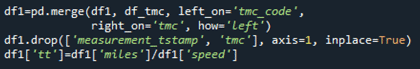Source: FHWA.

Figure 41. Screenshot. Code for calculating section travel time data.

8. Probe virtual method speed adjustment, if used:
• Prepare the subsections at any given time interval, by sorting the data by datetime and TMC order (“tmc_code,” “date” and “road_order”).
• Calculate cumulative time “tt_cum” and cumulative time step “tt_step.”
• Virtually simulate the vehicle movements by calculating the arrival time interval of a section based on the section travel times. The resulting arrival time interval (virtual) must be later than the original starting timestamp (snapshot).
• Sort by TMC code and datetime to replace the snapshot speeds by virtual simulated speeds.
• Ensure that the replacement only happens within the same TMC, and the process does not go beyond the last record of the dataset.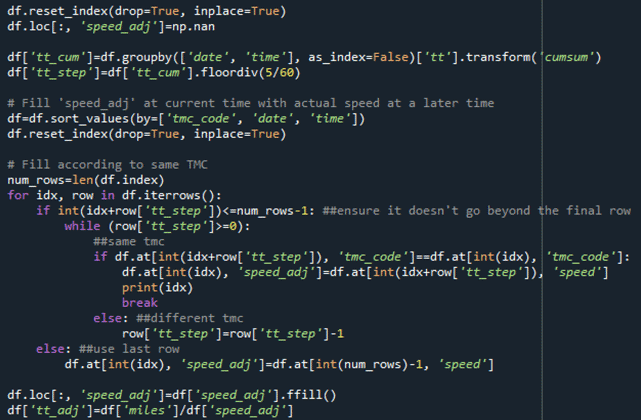Source: FHWA.

Figure 42. Screenshot. Code for creating travel times using the virtual probe method.

df.reset_index(drop=True, inplace=True) \\ df.loc[:, 'speed_adj']=np.nan \\ df['tt_cum']=df.groupby(['date', 'time'], asindex=False)['tt'], transform('cumsum') \\ df['tt_step']=df['tt_cum'], foordiv(5/60) \\ # Fill 'speed_adj' at current time with actual speed at a later time \\ df=df.sort.valyse(by=['tmc_code, 'date', 'time']) \\ df.reset_index(drop=True, inplace=True) \\ # Fill according to same TMC \\ num_rows=len(df.index) \\ for idx, row in df.iterrows(): \\ If int(idx+row['tt_step'])<=num_rows-1: ## ensure it doesn't go beyond the final row \\ While (row['tt_step]>=0: \\ ##same tmc \\ If df.at[int(idx+row['tt_step']), tmc_code]==df.at[int(idx), 'tmc_code']: df.at[int(idx), 'speed_adj']=df.at[int(indx+row['tt_step']), 'speed'] \\ print(idx) \\ break \\ else: ##different tmc \\ row['tt_step']=row[tt_step]-1 \\ else: ##use last row \\ df.at[int(idx), 'speed_adj']=df.at[int(num_rows)-1, 'speed'] \\ df.loc[:, 'speed_adj']=df['speed_adj'.ffile() \\ df['tt_adj']=df[miles]/df['speed_adj']
##### Data Aggregation: Produce Aggregated Facility-Level Data.
1. Aggregate section-level data to facility-level at each given datetime:
• Group data by datetime.
• Calculate the facility travel time and length by adding all section travel time (“tt” and length (“miles”) at each given time.
• Adjust facility travel time based on the ration of sum of the section lengths and facility length, in case missing section data exists.
• Calculate facility-level space-mean speeds.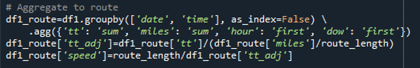Source: FHWA.

Figure 43. Screenshot. Code aggregating travel times to the section level.

df1_route= df1.groupby['date', 'time'], asindex=False) \ .agg9{'tt': 'sum', 'miles': 'sum', 'hour': 'first',' dow': 'first'}) \\ df1_route['tt_adj']=df1_route['tt']/(df1_route['miles']/route_length) \\ df1_route['speed']=route_length/df1_route['tt_ad']
2. Aggregate facility data to a specific time interval (e.g., 5-minute interval):
• Filter to nonholiday weekday dataset based on the “date” and “hour” fields.
• Group data by the specific time interval.
• Calculate mean facility travel times.
• Calculate corresponding facility speeds.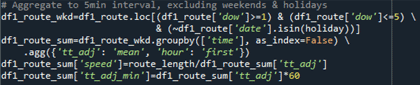Source: FHWA.

Figure 44. Screenshot. Code for aggregating travel time data to different temporal levels.

#### Creating Reliability Measures: Step-by-Step Calculation

##### PTI
1. Define peak periods based on the field context. Normally 7–9 a.m. and 4–6 p.m. should be used, but the peak periods can be shifted or extended based on field traffic conditions.
2. Use the facility-level travel time/speed dataset developed in the first step of the Data Aggregation section in this document.
3. Subset the dataset to weekday, weekend and holiday based on the datetime field.
4. Calculate free-flow travel time as 85th percentile speed on weekends and holidays during 6–10 a.m.
5. Calculate corresponding free-flow speeds.
6. Calculate PTI as the 95th percentile travel time during nonholiday weekday peak period (a.m. or p.m.) divided by free-flow travel time.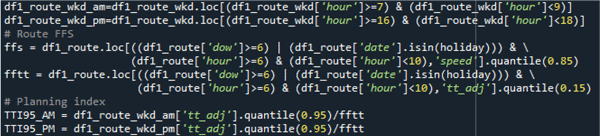Source: FHWA.

Figure 45. Screenshot. Code for creating free-flow speeds and travel times as well as the planning time index from aggregated travel time data.

df1_route_wkd_am=df1_route.wkd.loc[(df1_route_wkd['hour'}>=7 & (df1_route_wkd['hour']<9)] \\ df1_route_wkd_pm=df1_route.wkd.loc[(df1_route_wkd['hour'}>=16 & (df1_route_wkd['hour']<18)] \\ # Route FFS \\ ffs = df1_route_loc[((df1_route['dow'] >=6 | (df1_route['date'].isin(holiday))) & \ (df1_route['hour']>=6 & (df1_route['hour']<10), 'speed'].quantile(0.85) \\ fftt = df1_route_loc[((df1_route['dow'] >=6 | (df1_route['date'].isin(holiday))) & \ (df1_route['hour']>=6 & (df1_route['hour']<10), 'tt_adj'].quantile(0.15) \\ # Planning Time Index \\ TTI95_AM = df1_route_wkd_am['tt_adj']/quantile(0.95)/fftt \\ TTI95_PM = df1_route_wkd_pm['tt_adj']/quantile(0.95)/fftt

##### TTI80
1. Perform the same steps as step 1–5 in the PTI calculation.
2. Calculate TTI80 as the 80th percentile travel time during nonholiday weekday peak period (a.m. or p.m.) divided by free-flow travel time.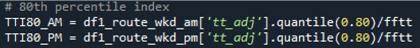Source: FHWA.

Figure 46. Screenshot. Code for creating the 80th percentile travel time index measure.

##### TTI50
1. Perform the same steps as step 1–5 in the PTI calculation.
2. Calculate TTI50 as the mean travel time during nonholiday weekday peak period (a.m. or p.m.) divided by free-flow travel time.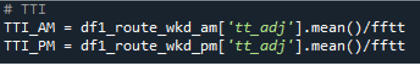Source: FHWA.

Figure 47. Screenshot. Code for creating the mean travel time index.

##### Semistandard Deviation
1. Perform the same steps as step 1–5 in the PTI calculation.
2. Calculate std as the standard deviation of travel time pegged to free-flow travel time (rather than the mean travel time) during nonholiday weekday peak period (a.m. or p.m.).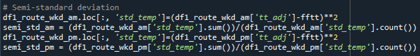Source: FHWA.

Figure 48. Screenshot. Code for creating the semistandard deviation.

df1_route_wkd_am.loc[:, 'std_temp'}=df1_route_wkd_am['tt_adj'}-fftt)**2 \\ semi_std_am = df1_route_wkd_am['std_temp].sum()/df1_route_wkd_am['std_temp'].count() \\ df1_route_wkd_pm.loc[:, 'std_temp'}=df1_route_wkd_pm['tt_adj'}-fftt)**2 \\ semi_std_am = df1_route_wkd_pm['std_temp].sum()/df1_route_wkd_pm['std_temp'].count()

##### Percent of Trips with Space-Mean Speed less than 30/45/50 Miles per Hour (pct_30, pct_45 and pct_50)
1. Perform the same steps as step 1–3 in the PTI calculation
2. Calculate pct_30/45/50 as the total number of observations with speeds below the specific thresholds divided by the total number of observations during nonholiday weekday peak period (a.m. or p.m.).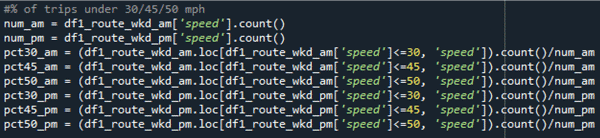Source: FHWA.

Figure 49. Screenshot. Code for creating the percent of trips operating at different speed thresholds.

num_am = df1_route_wkd_am['speed'].count() \\ num_pm = df1_route_wkd_pm['speed'].count() \\ pct_30_am = (df1_route_wkd_am.loc[df1_route_wkd_am['speed']<=30, 'speed]).count()/num_am \\ pct_45_am = (df1_route_wkd_am.loc[df1_route_wkd_am['speed']<=45, 'speed]).count()/num_am \\ pct_50_am = (df1_route_wkd_am.loc[df1_route_wkd_am['speed']<=50, 'speed]).count()/num_am \\ pct_30_pm = (df1_route_wkd_pm.loc[df1_route_wkd_pm['speed']<=30, 'speed]).count()/num_pm \\ pct_45_pm = (df1_route_wkd_pm.loc[df1_route_wkd_pm['speed']<=45, 'speed]).count()/num_pm \\ pct_50_pm = (df1_route_wkd_pm.loc[df1_route_wkd_pm['speed']<=50, 'speed]).count()/num_pm

##### PM3 LOTTR
1. Perform the same steps as step 1–3 in the PTI calculation.
2. Calculate LOTTR as the 80th percentile travel time divided by median travel time during nonholiday weekday peak period (a.m. or p.m.).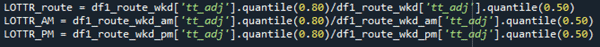Source: FHWA.

Figure 50. Screenshot. Code for creating level of travel time reliability metric (step 1).

##### PM3 Percent Length Reliable
1. Define peak periods based on the field context. Normally 7–9 a.m. and 4–6 p.m. should be used, but the peak periods can be shifted or extended based on field traffic conditions.
2. Use the section-level travel time/speed dataset developed in the Data Transformation section of this document.
3. Subset the section-level dataset to create nonholiday weekday and weekend datasets.
4. Assign four LOTTR periods based on the Federal PM3 hour definition.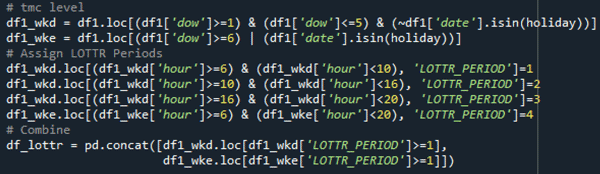Source: FHWA.

Figure 51. Screenshot. Code for creating level of travel time reliability metric (step 2).

# tmc level \\ df1_wkd = df1.loc[(df1['dow'}>=1) & (df1['dow']<= 5 & (~df1['date'].isin(holiday))] \\ df1_wke = df1.loc[(df1['dow'}>=6) & (df1['date']isin(holiday))] \\ # Assign LOTTR Periods \\ df1_wkd.loc[(df1_wkd['hour']>=6 & (df1_wkd['hour']<10, 'LOTTR_PERIOD]=1 \\ df1_wkd.loc[(df1_wkd['hour']>=10 & (df1_wkd['hour']<16, 'LOTTR_PERIOD]=2 \\ df1_wkd.loc[(df1_wkd['hour']>=16 & (df1_wkd['hour']<20, 'LOTTR_PERIOD]=3 \\ df1_wkd.loc[(df1_wke['hour']>=6 & (df1_wke['hour']<20, 'LOTTR_PERIOD]=4 \\ # Combine \\ df_lottr = pd.concat([df1_wkd.loc[df1_wkd['LOTTR_PERIOD']>=1, df1_wke.loc[df1_wke['LOTTR_PERIOD']>=1]])
5. Merge with the TMC definition dataset for the TMC length field “miles.”
6. Group the dataset by TMC and LOTTR period.
7. Calculate LOTTR values (80th percentile travel time divided by median travel time) for each TMC and LOTTR period.
8. Merge the dataset with the TMC definition data.
9. Determine the reliability of each TMC by comparing the LOTTR values with the 1.5 threshold value.
10. Calculate the ratio of total reliable TMC length to the facility length.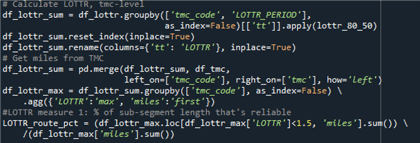Source: FHWA.

Figure 52. Screenshot. Code for creating level of travel time reliability metric (step 3).

#Calculate LOTTR, tmc-level \\ df_lottr_sum = df_lottr.groupby(['tmc_code', 'LOTTR_PERIOD], As)index=False)[['tt']].apply(lottr_80_50) \\ df_lottr_sum_reset_index(inplace=True') \\ df_lottr_sum_rename(columns={'tt}: 'LOTTR'}, inplace=True) \\ # Get miles from TMC \\ df_lottr_sum = pd.merge(df_lottr_sum, df_tmc, Left_on=['thmc_code'], as_index=False) \ .agg({'LOTTR': 'max', 'miles': 'first'}) \\ # LOTTR measure 1: %of sub-segment length that's reliable \\ LOTTR_route_pct = (df_lottr_max.loc[df_lottr_max['LOTTR']<1.5, 'miles].sum()) \ /(df_lottr_max['miles'}.sum()

This measure is not available for the trajectory data, as the trajectory data does not have subsections.

##### PMS System Reliability
1. Perform the same steps as step 1–4 in the PM3 percent length reliable calculation.
2. Aggregate the dataset to facility-level by group datetime.
3. Group the dataset by LOTTR period.
4. Calculate LOTTR values (80th percentile travel time divided by median travel time) for each LOTTR period.
5. Determine the reliability of the facility by comparing the LOTTR values with the 1.5 threshold value.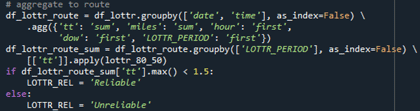Source: FHWA.

Figure 53. Screenshot. Code for creating system reliability measure.

# aggregate to route \\ df_lottr_route = df_lottr.groupby(['date', 'time'], as_index=False \ .agg({'tt': 'sum', 'miles': 'sum', 'hour': 'first', 'dow', 'first', 'LOTTR_PERIOD': 'first'}) \\ df_lottr_route_sum = df_lottr_route.groupby(['LOTTR PERIOD'], as_index=False) \ [['tt']].apply(lotte_80_50 \\ if df_lottr_sum['tt'].max() < 1.5: \\ LOTTR_REL = 'Reliable' \\ else: \\ LOTTR_REL = 'Unreliable'

24 Transportation Research Board of the National Academies of Sciences, Engineering, and Medicine. 2013. Analytic Procedures for Determining the Impacts of Reliability Mitigation Strategies. Report No. S2-L03-RR-1. Washington, DC: National Academy of Sciences. [Return to note 24]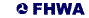United States Department of Transportation - Federal Highway Administration